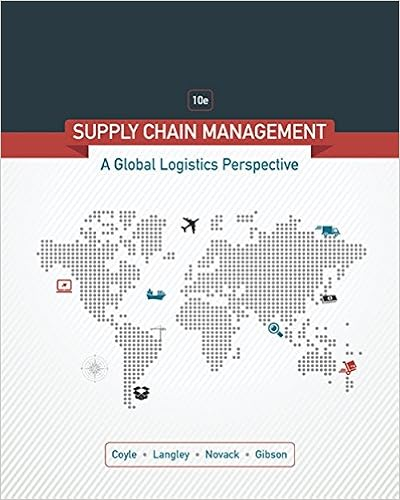# Between actual hours of input budgeted standard hours

• Homework Help
• 73
• 96% (750) 718 out of 750 people found this document helpful

This preview shows page 25 - 28 out of 73 pages.

##### We have textbook solutions for you!
The document you are viewing contains questions related to this textbook.The document you are viewing contains questions related to this textbook.
Chapter 6 / Exercise 1
Supply Chain Management: A Logistics Perspective
Coyle/LangleyExpert Verified
between actual hours of input, budgeted (standard) hours allowed for actual output, anddenominator level.An analysis of direct manufacturing labor will provide the data for actual hours of inputand standard hours allowed. One approach is to plug the known figures (designated by asterisks)into the analytical framework and solve for the unknowns. The direct manufacturing laborefficiency variance can be computed by subtracting \$9,640 from \$14,440. The complete pictureis as follows:Actual Costs IncurredActual Input× Budgeted RateFlexible Budget: Budgeted Input Allowed for Actual Output × Budgeted Rate(12,050 hrs. × \$16.80)\$202,440*(12,050 hrs. × \$16.00*)\$192,800(11,750 hrs. × \$16.00*)\$188,000* GivenDirect Labor calculationsActual input × Budgeted rate = Actual costs – Price variance= \$202,440 – \$9,640 = \$192,800Actual input = \$192,800 ÷ Budgeted rate = \$192,800 ÷ \$16 = 12,050 hours8-25\$9,640 U*Price variance\$4,800 UEfficiency variance\$14,440 U*Flexible-budget variance
##### We have textbook solutions for you!
The document you are viewing contains questions related to this textbook.The document you are viewing contains questions related to this textbook.
Chapter 6 / Exercise 1
Supply Chain Management: A Logistics Perspective
Coyle/LangleyExpert Verified
Budgeted input × Budgeted rate = \$192,800 – Efficiency variance= \$192,800 – \$4,800 = \$188,000Budgeted input = \$188,000 ÷ Budgeted rate = \$188,000 ÷ 16 = 11,750 hours = 10,000*× \$8.00 = \$117,6002.The calculations for total overhead are given below.Repair OverheadVariable overhead rate = \$64,000*÷ 8,000*hrs. = \$8.00 per standard labor-hour= \$197,600*– 10,000*× (\$8.00) = \$117,600If total overhead is allocated at 120% of direct labor-cost, the single overhead rate mustbe 120% of \$16.00, or \$19.20 per hour. Therefore, the fixed overhead component of the ratemust be \$19.20 – \$8.00, or \$11.20 per direct labor-hour.Let D = denominator level in input unitsBudgeted fixedoverhead rateper input unit=\$11.20=\$117,600DD=10,500 direct labor-hoursA summary 3-variance analysis for February follows:Actual Costs IncurredActual Inputs × Budgeted RateFlexible Budget: Budgeted Input Allowed for Actual Output × Budgeted RateAllocated:Budgeted InputAllowed forActual Output× Budgeted Rate\$249,000*\$117,600 + (12,050 × \$8.00)\$214,000\$117,600 + (\$8 × 11,750)\$211,600(11,750 hrs. × \$19.20)\$225,600*Known figureAn overview of the 3-variance analysis using the block format in the text is:8-26\$35,000 USpending variance\$2,400 UEfficiency variance\$14,000 F*Production-volume variance\$37,400 UFlexible-budget variance\$14,000 F*Production-volume variance
3-VarianceAnalysisSpendingVarianceEfficiencyVarianceProductionVolume VarianceTotal Overhead\$35,000 U\$2,400 U\$14,000 F3.The control of variable manufacturing overhead requires the identification of the costdrivers for such items as energy, supplies, equipment, and maintenance. Control often entailsmonitoring nonfinancial measures that affect each cost item, one by one. Examples are kilowattsused, quantities of lubricants used, and equipment parts and hours used. The most convincingway to discover why overhead performance did not agree with a budget is to investigate possiblecauses, line item by line item.Individual fixed manufacturing overhead items are not usually affected very much by day-to-day control. Instead, they are controlled periodically through planning decisions andbudgeting that may sometimes have horizons covering six months or a year (for example,management salaries) and sometimes covering many years (for example, long-term leases anddepreciation on plant and equipment).8-26 (35 min.)Production-volume variance analysis and sales volume variance.
•••